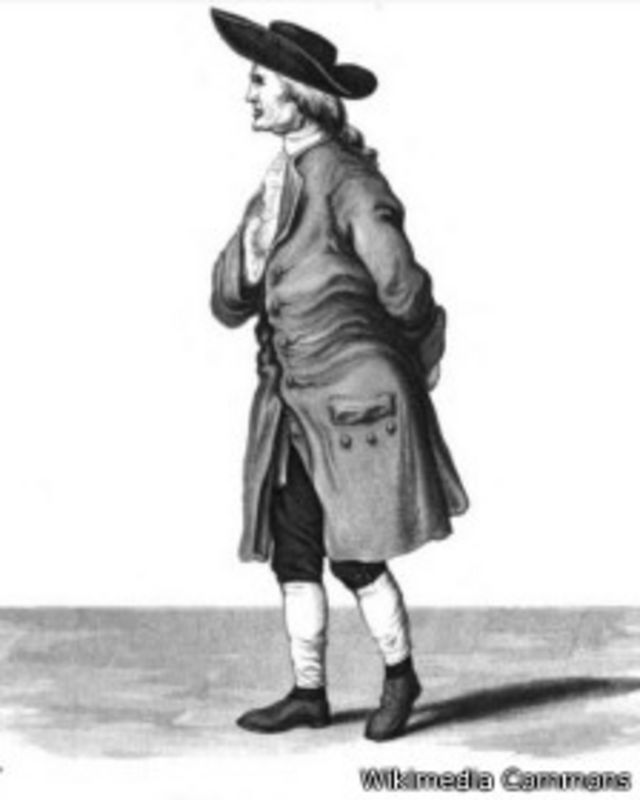# A lot of? Little bit? Do you want to know how much the Earth weighs?• Science
• BBC World, @bbc_ciencia

To know how much the Earth weighs, you have to take gravity into account and read this note to the end.

And how is gravity measured? The intensity of the force that keeps the Moon orbiting around the planet and us with our feet on the ground is determined by what physics calls “G”, the universal gravitational constant.caption, Henry Cavendish was the first to calculate the density and weight of the Earth.

“Newton’s law of gravity described the motion of the planets and moons with amazing precision, and what we call Newton’s constant of gravitation is what determines the scale, the magnitude of gravity,” Terry Quinn explained to the BBC, emeritus director of the International Bureau of Weights and Measures.

“Small-scale gravity is very weak, large-scale gravity is very, very large,” said the expert. Therefore, calculating a constant value was a challenge.